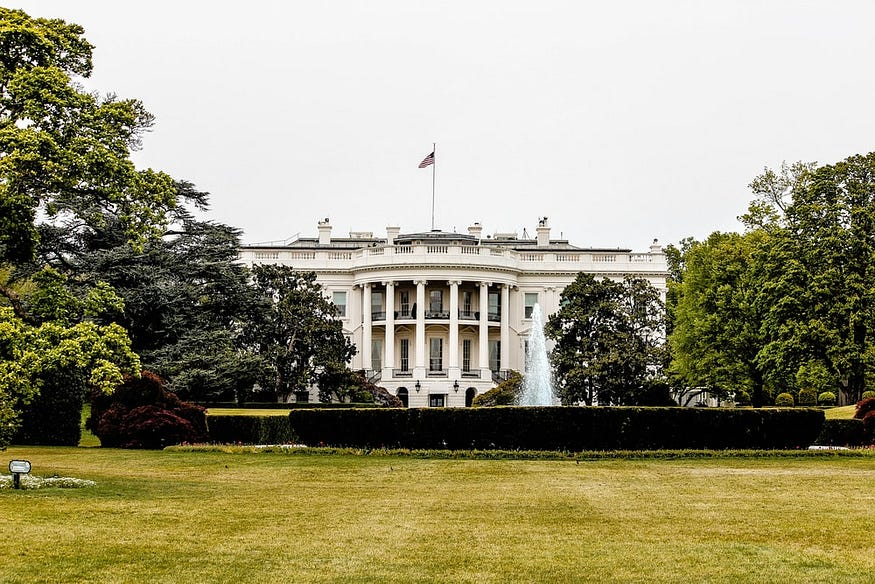Join thousands of students in our LangChain and Vector DBs in Production course, with over 50+ lessons and practical projects for FREE!.

## Publication# Finding Common Ground: US Presidents State Analysis

Last Updated on July 21, 2023 by Editorial Team

Originally published on Towards AI.

## Introduction

We aim to find any correlation between a US President and its state concerning other Presidents. We use data from different sources to form a dataset that comprises different US presidents and their home states with additional facts about the state. We pull data from multiple sources and combine it to form a comprehensive dataset. We also find the most common state and ultimately plot the results on a live interactive map.

• Python 3.8
• Pandas
• NumPy
• Folium
• Seaborn

## Data Preparation and Preprocessing

We start by preparing the data to comb the datasets from different sources and perform some basic preprocessing on it.

• We change the types
• Removed quotes and spaces
• Changed columns and combined two CSV files
`def load_data(path:str="/"): """ Loads the specified data using the path Args ------- path: str (where president_timelines.csv and president_states.csv reside) Returns -------- The combined dataframe """ df_time = pd.read_csv("president_timelines.csv") df_states = pd.read_csv("president_states.csv") # Setting columns as specified by the dataframe df_time.columns = ["Index", "Name", "Birth", "Death", "TermBegin", "TermEnd"] df_states.columns = ["Name", "Birth State"] president_names = df_time.Name print(f"Total number of presidents: {len(president_names)}") president_states = df_states["Birth State"] print(f"Total states: {len(president_states)}") df = pd.DataFrame({"name":president_names, "states":president_states}) # changing type df.name = df.name.astype(str) df.states = df.states.astype(str) # removing whitespaces df['states'] = df['states'].apply(lambda x: x.strip()) # removing quotes df.states = df.states.apply(lambda x: x.replace('"',"")) df.name = df.name.apply(lambda x: x.replace('"',"")) return df`

We also change the types of the columns to be able to use it later.

## Finding Common Ground

Now, we start by writing a function that can find all the common states.

• We start by creating a map of state and name
• We also create a reverse index for the same
• We use the collections. Counter class to get the most common count and find the state using the reverse lookup index
• We find the top 5 states and print it
`def common(show_counter=False, show_prez=False): """ Find the common data points in the dataset """ from collections import Counter mapping_state_name = {state:name for state,name in zip(df['states'].unique(), df['name'].unique())} df['states'] = df['states'] counter = Counter(df['states'].values) counter_vice_versa = {counter[key]: key for key in counter.keys()} max_counter = max(counter) count = sorted(counter.values()) second_largest_count = count[-2] print(f"Maximum occured state: {max_counter}.") print(f"Second largest state: {counter_vice_versa[second_largest_count]}") print(f"Third largest state: {counter_vice_versa[count[-3]]}") print(f"Fourth largest state: {counter_vice_versa[count[-4]]}") print(f"Fifth largest state: {counter_vice_versa[count[-5]]}") # presidents with the max_counter if show_prez: for name, state in zip(df['name'], df['states']): if state == max_counter: print(f"President with {max_counter} was {name}.") if show_counter: print(counter.keys())common(False, True)`

## Another way of finding common ground using a matrix-based approach

We also use a different technique to get the common states. We plot a heatmap to get the results in a visually appealing way.

`matrix_based = df.groupby("name")['states'].value_counts().unstack().fillna(0)sns.heatmap(matrix_based, vmax=1, vmin=0, cmap="gray")plt.title("Finding common ground")`
• Here you can see, for every president in a particular state, we have 1.0, and if a president is repeated, then we have two or 1s in a column
• This is a nice representation of how the presidents are distributed statewide
• This heatmap shows that values that are 0 have black color and white denotes 1.0

## Further analysis using data from another source

We will be using data from the CDC for each state to find some interesting insights.

`def load_data_from_cdc(save=False): """ Load data from CDC """ # reading the html table at index 0 df_states_fact = pd.read_html("https://www.cdc.gov/nchs/fastats/state-and-territorial-data.htm") # changing column (for join) df_states_fact['states'] = df_states_fact['State/Territory'] # removing old column df_states_fact.drop(columns=['State/Territory'], inplace=True) merged = pd.merge(df, df_states_fact) # Save the csv if save: merged.to_csv(index=False) merged.Births = merged.Births.astype(float) merged['Fertility Rate'] = merged['Fertility Rate'].astype(float) merged['Death Rate'] = merged['Death Rate'].astype(float) return mergeddf_merged = load_data_from_cdc()`
• The Correlation matrix does not show much of a relationship between the data points.
• Let’s try to change the name and states into numeric values and then try to perform correlation
`def apply_transformations(df: pd.DataFrame): """ Apply transformation on states and name """ # mapping of states and names mapping_states = {state: index for index, state in enumerate(df['states'])} mapping_name = {name: index for index, name in enumerate(df['name'])} df.states = df.states.apply(lambda x: mapping_states[x]) df.name = df.name.apply(lambda x: mapping_name[x]) return dfdf_transformed = apply_transformations(df_merged)`

## Some interesting plots

Let us plot some interesting datapoints

## Plotting maps

To better understand the data, let us plot a map with all the marked presidents. To solve this, we combine a latitude and longitude dataset with our US Presidents dataset.

`map_osm = folium.Map(width=500,height=500,location=[37.0902, -95.7129], zoom_start=3)df_final.apply(lambda row:folium.CircleMarker(location=[row["Latitude"],  row["Longitude"]]).add_to(map_osm), axis=1)`

We then plot our map using Folium

## Conclusion

• On analyzing the data, we found some very fascinating insights
• We tried to combine data from different sources to get our answers
• We used different ways to visualize our data
• We found the most common states
• We could have performed better if we had access to a wide variety of data about the state, such as education, income, etc

Join thousands of data leaders on the AI newsletter. Join over 80,000 subscribers and keep up to date with the latest developments in AI. From research to projects and ideas. If you are building an AI startup, an AI-related product, or a service, we invite you to consider becoming a sponsor.

Published via Towards AI